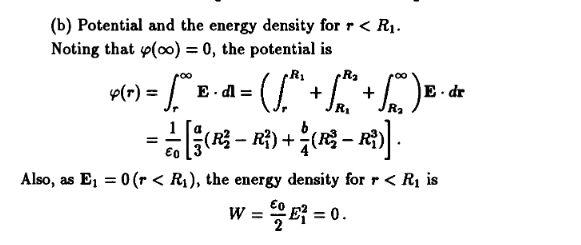# Help with electrostatics problem (spherical shell charge distribution)

• sroot

#### sroot

Homework Statement
A static electric charge is distributed in a spherical shell of inner radius R1 and outer radius R2. The electric charge density is given by ρ=a+br, where r is the distance from the centre, and zero everywhere else.
(i) Find an expression for the electric field everywhere in terms of r.
(ii) Find expressions for the electric potentials and energy density for r < R1. Take the potential to be zero at r→∞.
Relevant Equations
ρ=a+br

Homework Statement:: A static electric charge is distributed in a spherical shell of inner radius R1 and outer radius R2. The electric charge density is given by ρ=a+br, where r is the distance from the centre, and zero everywhere else.
(i) Find an expression for the electric field everywhere in terms of r.
(ii) Find expressions for the electric potentials and energy density for r < R1. Take the potential to be zero at r→∞.
Relevant Equations:: ρ=a+br

According to my professor, the solution in this book (pages 20-21) for item (ii) is wrong: https://www.u-cursos.cl/usuario/754...roblems_and_Solutions_on_Electromagnetism.pdf
Welcome to PF.

Can you please just take screenshots of the pages that you want to discuss? Asking us to download the whole book PDF file is a bit too much. And please explain what is confusing you about the solution. Thank you.

Welcome to PF.

Can you please just take screenshots of the pages that you want to discuss? Asking us to download the whole book PDF file is a bit too much. And please explain what is confusing you about the solution. Thank you.

This is the solution for item (b). According to the professor, the integral is completely off. I can't understand why.Did you carry out the integrations? What did you get for the results of the individual integrals?

It looks like the solution asserts that the electric field is the same in all regions by showing it factored out of the integrals. That is simply not true. I started doing the integrals but I quit before I got to the bottom line when it became obvious that my expression would not simplify to the answer shown above.

I interpreted "The electric charge density is given by ρ=a+br, where r is the distance from the centre, and zero everywhere else" to mean that there is charge only in the region ##R_1\leq r \leq R_2## "everywhere else" being outside this region. Otherwise why bother mentioning a shell?

Also, it is misleading (but not an error) to write the left hand side as ##\varphi (r)## when it is actually independent of ##r##.

Last edited:
•berkeman